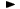ADVFN HomeHelpFinancialsDeeper AnalysisINVESTMENT RATIOS - Market Value AnalysisTobin's Q Ratio

# Tobin's Q Ratio

Tobin's Q Ratio, is the market value of a company's assets divided by their replacement value. Replacement value being the current cost of replacing the firms assets. This ratio is named after Nobel Economics Laureate James Tobin of Yale University. He hypothesized that the combined market value of all the companies on the stock market should be about equal to their replacement costs. In other words, the ratio of all the combined stock market valuations to the combined replacement costs should be around one. The formula is the following:

market cap. / average total assets